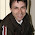Solving the GDP equation: Macroeconomics and the GDP equation, Y=C+I+G+NX - FreeEconHelp.com, Learning Economics... Solved!

6/26/11

When looking at the basic macroeconomy, we need to know what components make up GDP (Gross Domestic Product).  The most basic equation for representing GDP is the following:

Y=C+I+G+NX

where Y is GDP
C is consumer spending
I is investment
G is government spending
and NX is net exports.

This means the GDP of an economy (or the total value of all of the final outputs),
is equal to the amount bought by consumers (C), the amount bought by the government (G), the amount bought by businesses (I), and the net bought by the rest of the world (NX).  NX will be positive only if we export a higher value of goods than we import, and it will be negative if we import a higher value of goods than we export.

This equation shows why it is important to have high levels of consumer spending, government spending, business investment, and exports.  Each of these components contribute equally to GDP which is a standard measure for measuring prosperity for a nation.

For example, if consumer spending and investment go down (like in a recession), than GDP will also go down.  If the government wants to avoid this, they can increase their own spending, by raising G, or devalue their currency or take other approaches to try and raise their exports (and therefore NX).

Lets do some examples.  Find G if:
GDP=1,200
Consumer spending=800
Investment=200
and net exports=-100

All we have to do is recognize where these values go, plug in, and solve for G:

1,200=800+200+G-100
or G=1,200-800-200+100=300
So in this problem government spending is equal to 300.

Solve for investment (I) if:
GDP=40
Consumer Spending=25
Government spending=5
and net exports=5

so:

40=25+I+5+5
or I=40-25-5-5=5

So investment is equal to 5.

Sometimes you will get huge numbers in the trillions or billions but don't let that confuse you.  Just remember your basic:
GDP=C+I+G+NX equation and you will be good.

HINT: If you are having trouble remember the equation, remember the acronym, CIGS (like cigarettes), for C I G S, consumer spending, investment spending, and government spending.  If you are in a class that also requires NX, then you will just have to remember that on your own ;).

1 comment:

1.cool lesson!!!